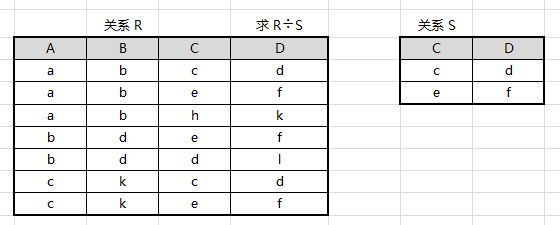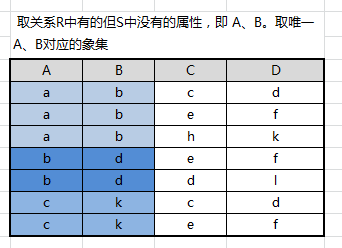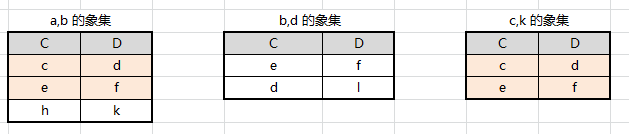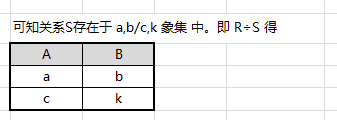关系代数的基本运算

关系代数运算符

$∪$ Union
$−$ Difference
$∩$ Intersection
$×$ 笛卡尔积 Cartesian Product

$>$ 大于
$≥$ 大于等于
$<$ 小于
$≤$ 小于等于
$=$ 等于
$≠$ 不等于

$σ$ 选择 Selection
$π$ 投影 Projection
$⋈$ 链接 Join
$÷$ Division

$∧$
$∨$
$¬$

5 种基本的关系代数运算

并（Union）

$$R∪S=\{t|t∈R∨t∈S\}$$

差（Difference）

$$R−S=\{t|t∈R∨t∉S\}$$

广义笛卡尔积（Extended Cartesian Product）

$$R×S=\{t|t=<(t^n,t^m)∧t^n∈R∧t^m∈S\}$$

$(t^n,t^m)$ 表示元素 $t^n$ 和 $t^m$ 拼接成的一个元组

投影（Projection）

$$π_A(R)=\{t[A]|t∈R\}$$

选择（Selection）

$$σ_F(R)=\{t|t∈R∧F(t)=True\}$$

实例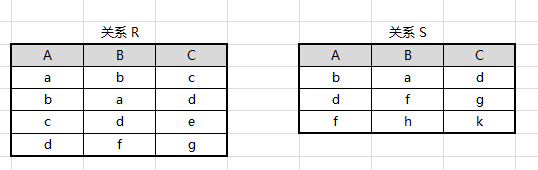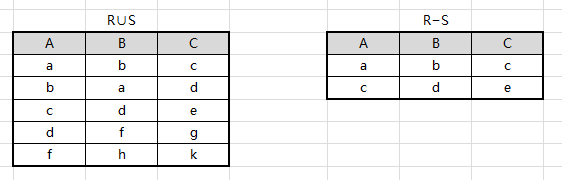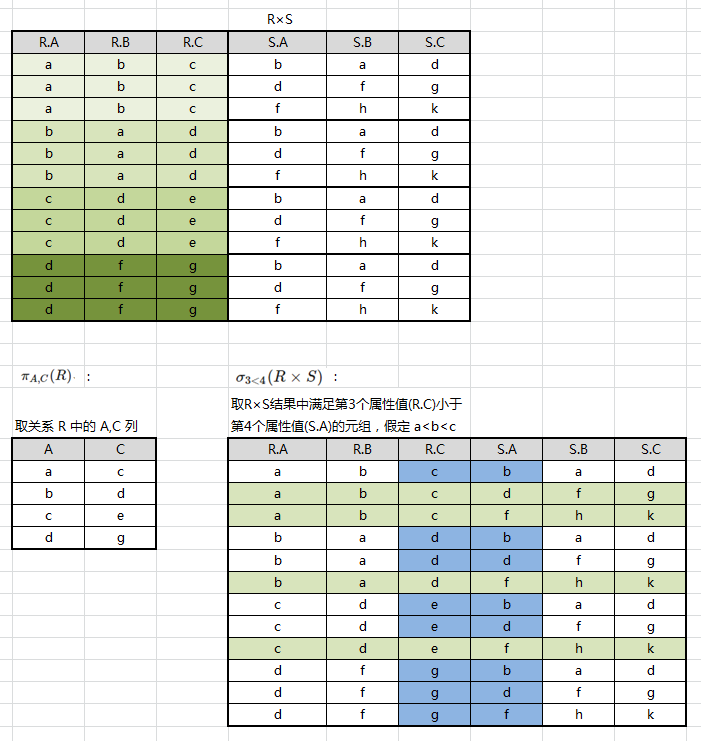扩展的关系代数运算

交（Intersection）

$$R∩S=\{t|t∈R∧t∈S\}$$

链接（Join）

θ 链接

$$R \Join_{XθY} S = σ_{XθY}(R×S)$$

XθY 为链接的条件，θ 是比较运算符，X 和 Y 分别为 R 和 S 上度数相等且可比的属性组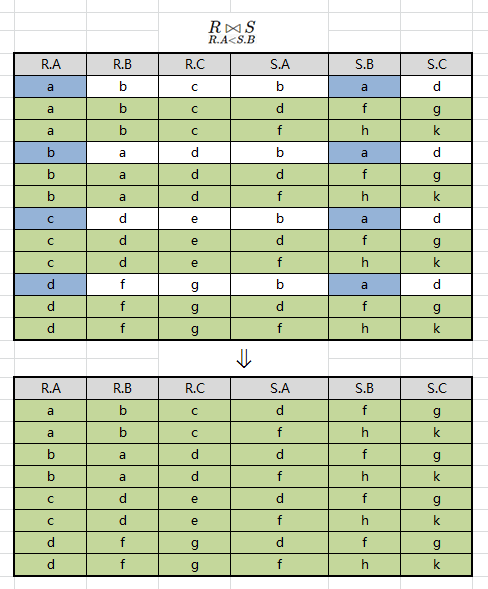自然链接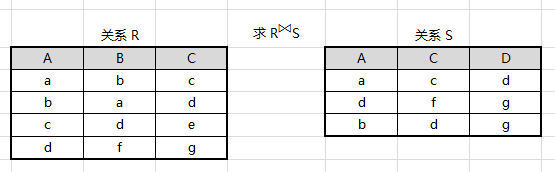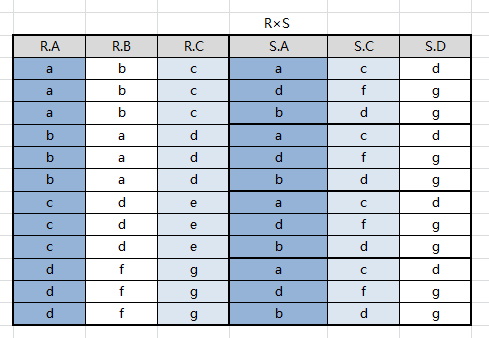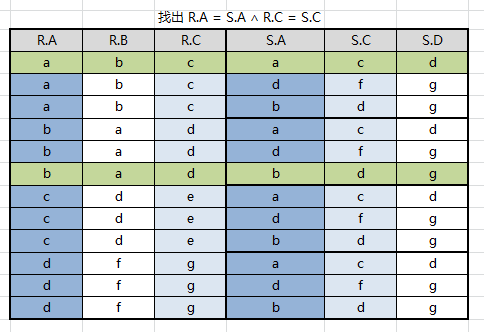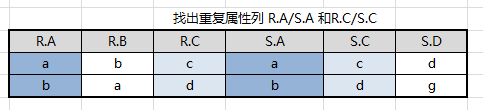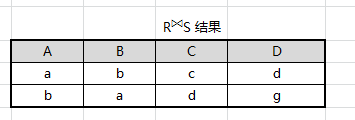除（Division）